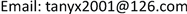“嫦娥三号”软着陆轨道设计与控制策略<sup> </sup>1. 引言

2. 确定嫦娥三号所需开普勒轨道中近月点和远月点的参数2.1. 建模分析

2.2. 动力学模型的建立与求解

{ 1 2 r A v A = 1 2 r B v B 1 2 m v A 2 − m g A r A = 1 2 m v B 2 − m g B r B

g A = G M M r A 2 , g B = G M M r B 2

2.3. 近月点与远月点位置的确定

44.12 ∘ − 12.6397 ∘ = 31.4803 ∘

3. 软着陆六个阶段的最优控制策略3.1. 建模分析

min z = ∫ t 0 t f u x 2 ( t ) + u y 2 ( t ) v e d t

s .t     d 2 x d t 2 = G M M x 2 + y 2 sin α ( t ) + u x ( t ) m ( t ) ,

d 2 y d t 2 = G M M x 2 + y 2 cos α ( t ) + u y ( t ) m ( t ) ,

d m d t = u x 2 ( t ) + u y 2 ( t ) v e ,

m ( t 0 ) = m 0 , x ( t 0 ) = x 0 , y ( t 0 ) = y 0 , d x d t ( t 0 ) = v x 0 , d y d t ( t 0 ) = v y 0 ,

m ( t f ) = m f , x ( t f ) = x f , y ( t f ) = y f , d x d t ( t f ) = v x f , d y d t ( t f ) = v y f .

3.2. 模型的建立与求解3.2.1 主减速阶段的模型

u x 2 ( t ) + u y 2 ( t ) = 7500

m ( t 0 ) = 2400 , x ( t 0 ) = 0 , y ( t 0 ) = y 0 1752013 , d x d t ( t 0 ) = 1692.7 , d y d t ( t 0 ) = 0 ,

x 2 ( t f ) + y 2 ( t f ) = 1737373 ,   ( d x d t ( t f ) ) 2 + ( d y d t ( t f ) ) 2 = 57.

Step 1. 将7个取值范围给定的优化参量按一定的浮点数编码排列在一起成为一个个体，随机产生2000个这样的个体作为初始种群；

Step 2. 通过编写的适应函数计算每一个个体的性能指标；

Step 3. 使用轮盘赌法作为选择算子并对这N个个体进行排序；

Step 4. 选择出若干个性能指标取值较优的个体保留，并将其遗传到下一代；

Step 5. 将个体随机两两配对，按照既定的交叉概率进行交叉操作；

Step 6. 对每一个个体中的每一个参数，按照既定的变异概率进行编译操作；

Step 7. 若满足收敛条件则输出最优解，否则继续进行编码、评价、选择、交叉和变异等操作。

3.2.2. 快速调整阶段的控制策略

3.2.3. 粗避障阶段的最优控制策略

3.2.4. 精避障阶段的模型

3.2.5. 缓速下降阶段的控制策略

3.2.6. 自由下降阶段的控制策略

4. 结论

2015年度湖南省大学生研究性学习和创新性实验计划项目，项目编号：201510536011。